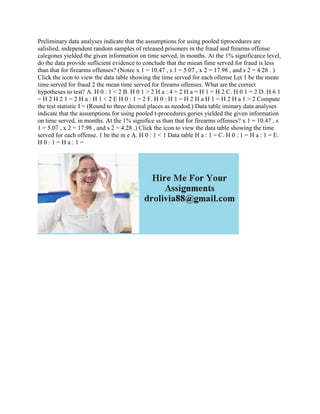PublicitéProchain SlideShareProblem 1-50 (Algo) Cost Data for Managerial Purposes-Finding Unknowns.docx
Chargement dans ... 3
1 sur 1
Publicité

### Preliminary data analyses indicate that the assumptions for using pool.docx

1. Preliminary data analyses indicate that the assumptions for using pooled tiprocedures are salislied. independent random samples of released prisoners in the fraud and frearms offense calegones yielded the given information on time served, in months. At the 1% significance level, do the data provide suflicient evidence to conclude that the miean fime served for fraud is less than that for firearms offenses? (Notec x 1 = 10.47 , s 1 = 5.07 , x 2 = 17.98 , and s 2 = 4.28 . ) Click the icon to view the data table showing the time served for each ollense Let 1 be the mean time served for fraud 2 the mean time served for fireams oflenses. What are the correct hypotheses to test? A. H 0 : 1 < 2 B. H 0 1 > 2 H a : 4 = 2 H a = H 1 = H 2 C. H 0 1 = 2 D. H 6 1 = H 2 H 2 1 = 2 H a : H 1 < 2 E H 0 : 1 = 2 F. H 0 : H 1 = H 2 H a H 1 = H 2 H a 1 > 2 Compute the test statistic I = (Round to three decmal places as neodod.) Data table iminary data analyses indicate that the assumptions for using pooled t-procedures gories yielded the given information on time served, in months. At the 1% significe ss than that for firearms offenses? x 1 = 10.47 , s 1 = 5.07 , x 2 = 17.98 , and s 2 = 4.28 .) Click the icon to view the data table showing the time served for each offense. 1 be the m e A. H 0 : 1 < 1 Data table H a : 1 = C. H 0 : 1 = H a : 1 = E. H 0 : 1 = H a : 1 =
Publicité